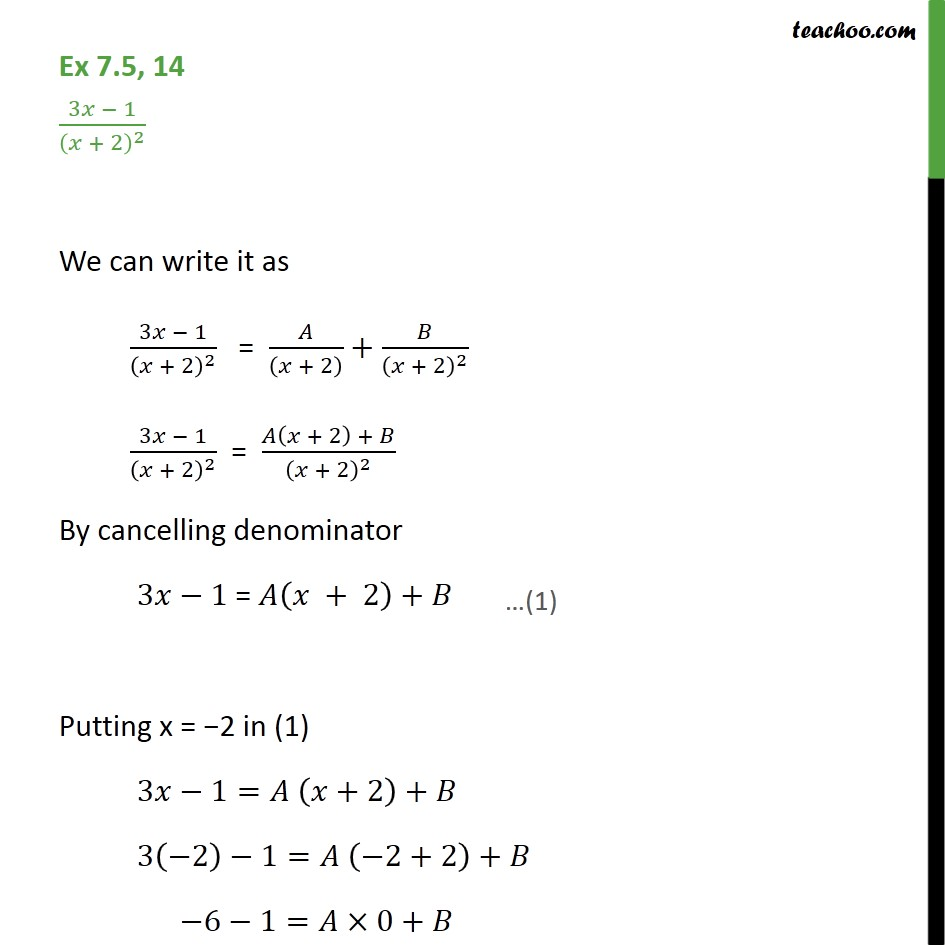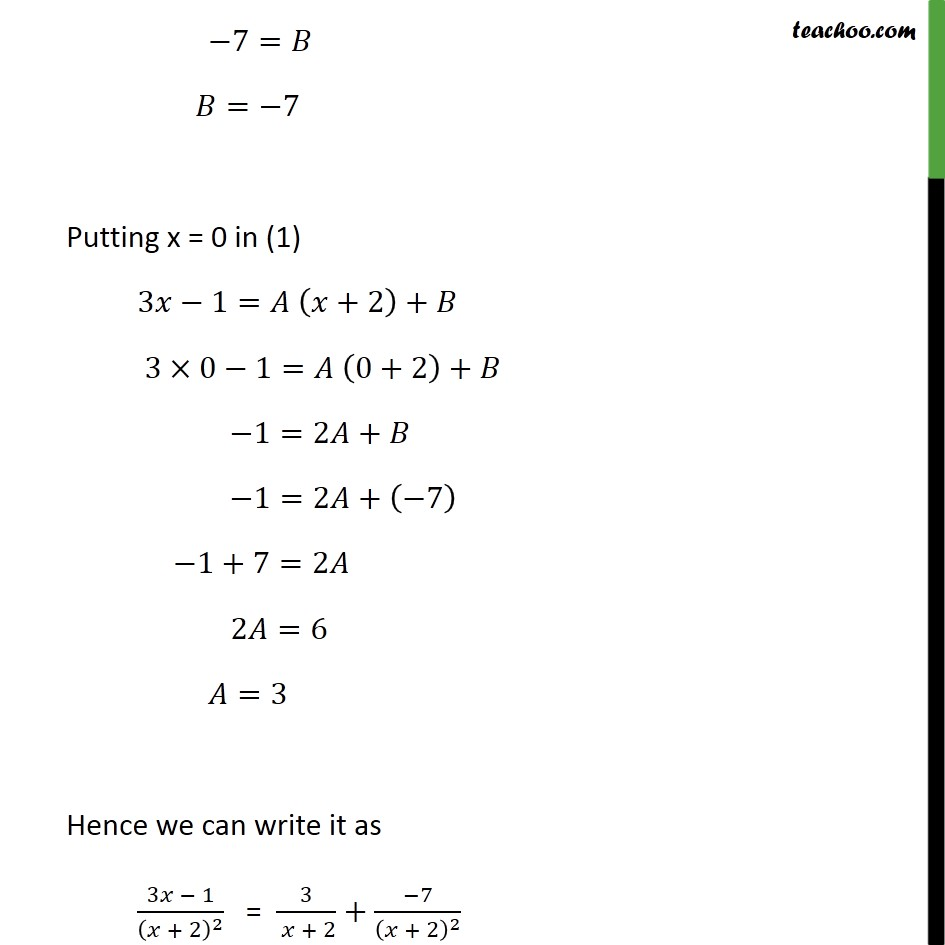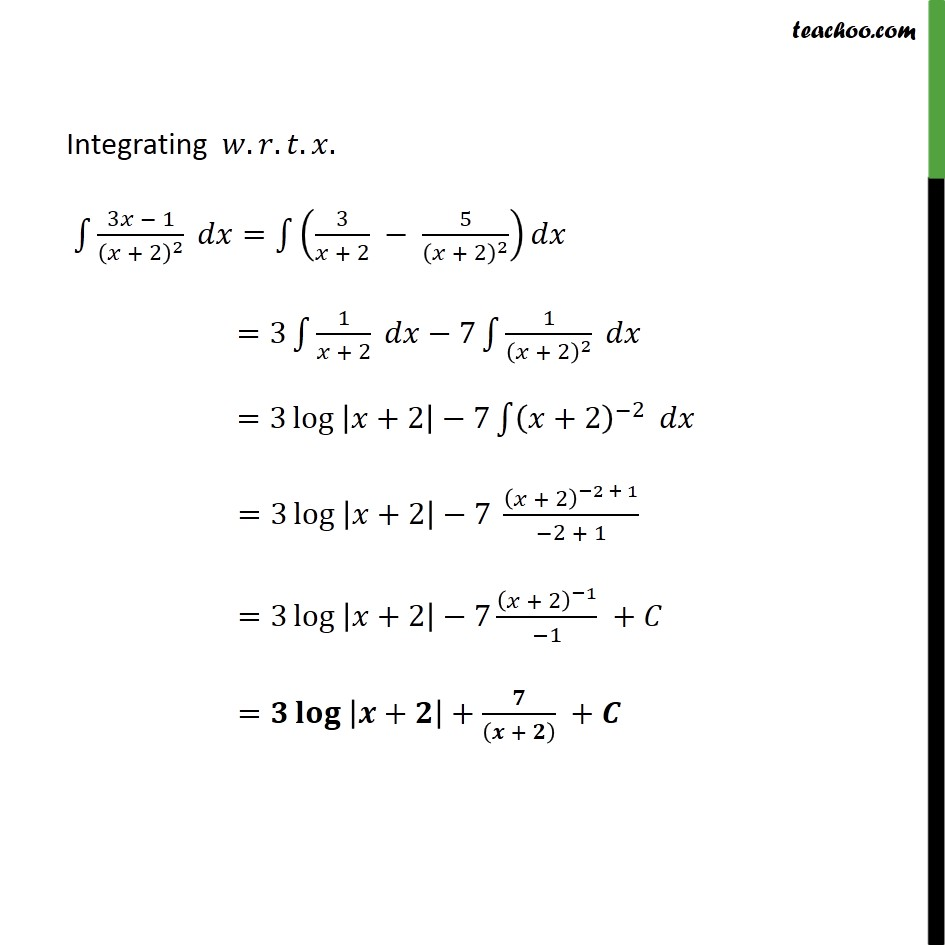Integration by partial fraction - Type 2

Chapter 7 Class 12 Integrals
Concept wiseLearn in your speed, with individual attention - Teachoo Maths 1-on-1 Class

### Transcript

Ex 7.5, 14 (3𝑥 − 1)/(𝑥 + 2)^2 We can write it as (3𝑥 − 1)/(𝑥 + 2)^2 = 𝐴/((𝑥 + 2) )+𝐵/(𝑥 + 2)^2 (3𝑥 − 1)/(𝑥 + 2)^2 = (𝐴(𝑥 + 2) + 𝐵)/(𝑥 + 2)^2 By cancelling denominator 3𝑥−1 = 𝐴(𝑥 + 2)+𝐵 Putting x = −2 in (1) 3𝑥−1=𝐴 (𝑥+2)+𝐵 3(−2)−1=𝐴 (−2+2)+𝐵 −6−1=𝐴×0+𝐵 −7=𝐵 𝐵=−7 Putting x = 0 in (1) 3𝑥−1=𝐴 (𝑥+2)+𝐵 3×0−1=𝐴 (0+2)+𝐵 −1=2𝐴+𝐵 −1=2𝐴+(−7) −1+7=2𝐴 2𝐴=6 𝐴=3 Hence we can write it as (3𝑥 − 1)/(𝑥 + 2)^2 = 3/( 𝑥 + 2)+(−7)/(𝑥 + 2)^2 Integrating 𝑤.𝑟.𝑡.𝑥. ∫1▒(3𝑥 − 1)/(𝑥 + 2)^2 𝑑𝑥=∫1▒(3/(𝑥 + 2) − 5/(𝑥 + 2)^2 ) 𝑑𝑥 =3∫1▒〖1/(𝑥 + 2) 〗 𝑑𝑥−7∫1▒〖1/(𝑥 + 2)^2 〗 𝑑𝑥 =3 〖log 〗⁡|𝑥+2|−7∫1▒〖(𝑥+2)^(−2) 〗 𝑑𝑥 =3 〖log 〗⁡|𝑥+2|−7 (𝑥 + 2)^(−2 + 1)/(−2 + 1) =3 〖log 〗⁡|𝑥+2|−7 (𝑥 + 2)^(−1)/(−1) +𝐶 =𝟑 〖𝐥𝐨𝐠 〗⁡|𝒙+𝟐|+𝟕/((𝒙 + 𝟐) ) +𝑪